## Generalized velocity-dependent seislet transform

The seislet framework was defined by Fomel and Liu (2010). It is based on the discrete wavelet transform and different data patterns, such as slopes or frequencies. In plane-wave construction (Fomel, 2010; Fomel and Guitton, 2006), a seismic trace is predicted from its neighbors by following locally varying slopes of seismic events. It has been used for designing PWD-seislet transforms. Liu et al. (2015) proposed a velocity-dependent (VD) slope as the pattern in VD-seislet transform, where the normal moveout (NMO) equation serves as a bridge between local slopes and scanned NMO velocities. Seislet transforms with slope patterns show different characteristics compared to wavelet transform. Wavelet transforms involve no special pattern and sample shift is treated as an elementary prediction. Compared with PWD-seislet transform that predicts local plane waves, VD-seislet transforms involve the traces in common-midpoint (CMP) gather and predict elements along a time-distance curve.

The general guidelines of the lifting scheme (Sweldens and Schröder, 1996) can be followed for the discrete wavelet transform to define seislet transforms. The basic predictionand updateoperators for a simple seislet transform are shown as follows (Fomel and Liu, 2010):(1)(2)

where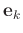is the even components of data at the-th transform scale,is the residual difference between the odd component of data and its prediction from the even component at the-th transform scale.andare operators that predict a component from its left and right neighbors according to different patterns. Equations 1 and 2 are defined by modifying Cohen-Daubechies-Feauveau (CDF) 5/3 biorthogonal wavelet construction (Cohen et al., 1992). A higher-order seislet transform can also be defined by applying biorthogonal wavelets twice with different lifting operator coefficients (Lian et al., 2001). For different types of slope-based seislets, the corresponding operator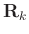, which is related to different slope patterns by plane-wave construction (Fomel, 2010), must be defined.

Liu et al. (2015) used the classical hyperbolic model of reflection moveout at near offset to define seismic local slopes(3)

where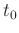is the zero-offset traveltime,is the corresponding traveltime recorded at offset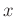, andis the stacking or root mean square (RMS) velocity obtained through a standard velocity scan.

For more general cases, the shifted hyperbola NMO equation (Malovichko, 1978; Castle, 1994; Fomel and Grechka, 2001) can be used to obtain more accurate slopes in some situations, such as large-offset gather or VTI media. The nonhyperbolic NMO equation is shown as follows:(4)

whereis the parameter describing heterogeneity. Equation 4 is reduced to equation 3 when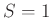.

The generalized velocity-dependent (VD) slopescan be calculated by:(5)

After the generalized velocity-dependent (VD)-slope pattern of seismic data is calculated, pattern-based operatorscan be designed through plane-wave construction, which guarantees representation of nonhyperbolic primaries by the generalized VD-seislet transform. When the generalized VD-seislet transform is applied to a CMP gather, the predictable reflection information is compressed to large coefficients at small scales. The sparse characteristic of the generalized VD-seislet transform is suitable for reconstructing missing data in the inverse problem framework.

2019-05-06